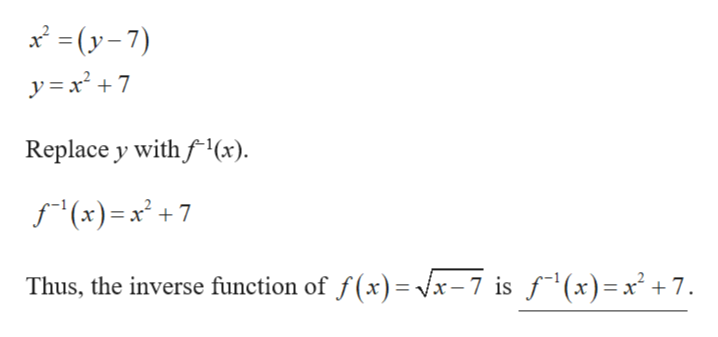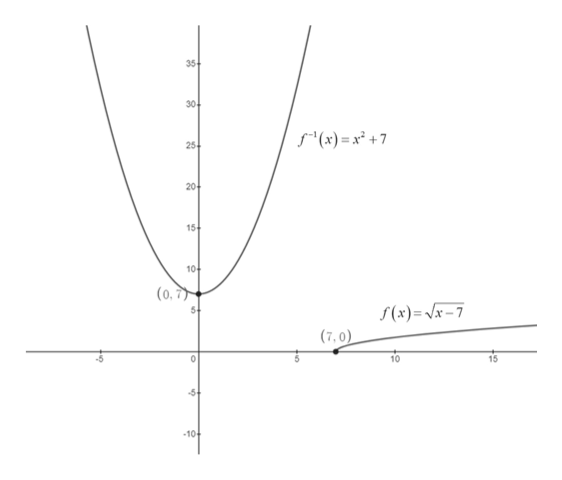# Given the function f(x) vx-7,(a) Find f(b) Graph f andf in the same rectangular coordinate system.(c) Use interval notation to give the domain and the range of f and f(x).-1()y)(Hint: To solve for a variable involving an nth root, raise both sides of the equation to the nth power,c) State the domain and range of f and f using interval notation.Select the corect choice below and fill in the answer box to complete your choice.(Type your answer in interval notation.)isO A. The domain of f, which is equal to the range of f1isD.The domain of f. which is equal to the domain of fSelect the correct choice below and fill in the answer box to complete your choice.(Type your answer in interval notation.)1Click to select and enter your answer(s).searchhpHEWLE T T PACK ARDinsprt scholl12fef614delet5&7%54backs6RTYUP**K LFGH00

Question
check_circle

Step 1

(a)

It is given that the function f(x) = √(x – 7).

Obtain the inverse of the function as follows.

Substitute y for f(x) in f(x) = √(x – 7).

y = √(x – 7)

Interchange x and y.

x = √ (y – 7)

Take square on both sides,

x2 = (y – 7)

Step 2

Solve the equation to obtain y.help_outlineImage Transcriptionclose=(y-7) Replace y with f(x) f(x)= x +7 Thus, the inverse function of f(x) Vx-7 is f1(x)=x + 7 fullscreen
Step 3

Use online graphing calculator to graph the func...help_outlineImage Transcriptionclose35 30 f" 7+ 'א ((א - . 25 20 15+ 10- (0,7 5 (x)= Vx-7 (7,0) 10 10+ fullscreen

### Want to see the full answer?

See Solution

#### Want to see this answer and more?

Solutions are written by subject experts who are available 24/7. Questions are typically answered within 1 hour.*

See Solution
*Response times may vary by subject and question.
Tagged in

### Other